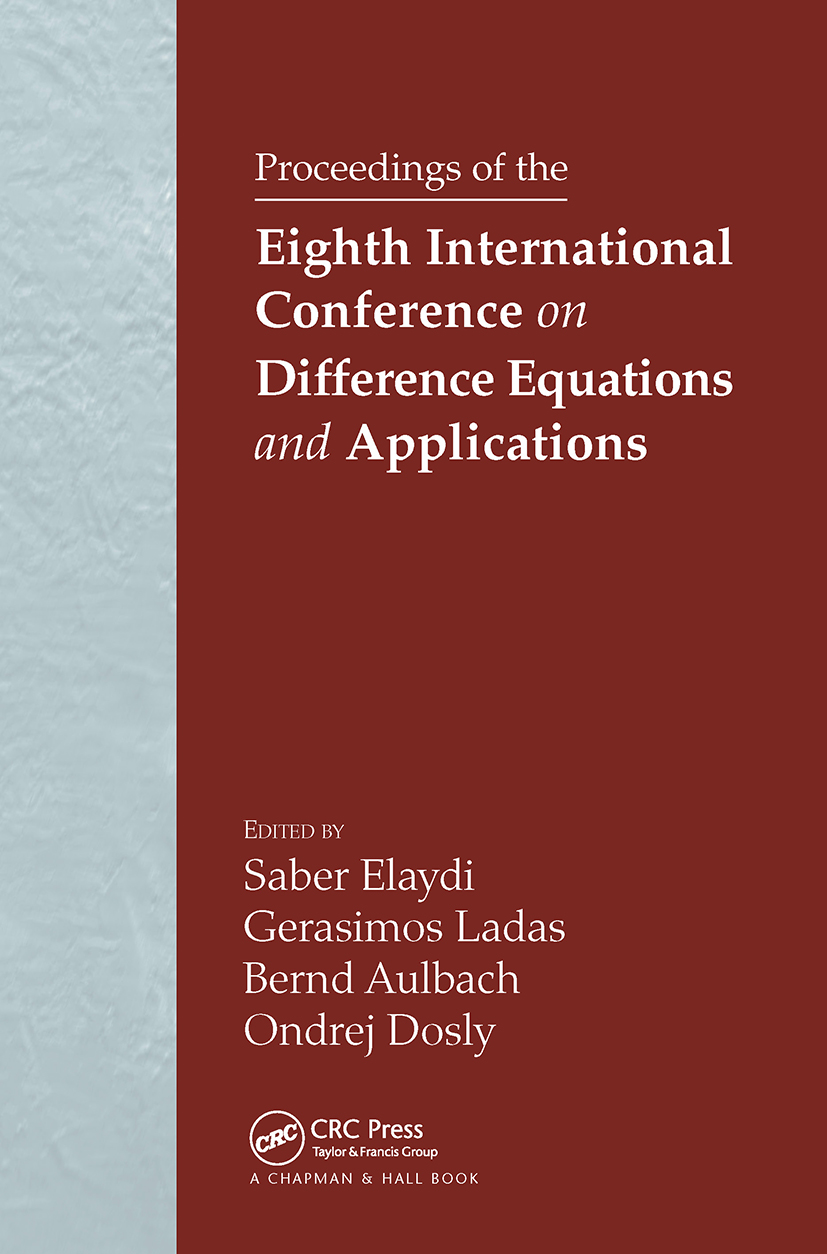Proceedings of the Eighth International Conference on Difference Equations and Applications

1st Edition

Chapman and Hall/CRC

304 pages | 15 B/W Illus.

Hardback: 9781584885368
pub: 2005-04-29
Currently out of stock
\$275.00
x
eBook (VitalSource) : 9780429137976
pub: 2005-04-29
from \$28.98

FREE Standard Shipping!

Description

The Eighth International Conference on Difference Equations and Applications was held at Masaryk University in Brno, Czech Republic. This volume comprises refereed papers presented at this conference. These papers cover all important themes, conjectures, and open problems in the fields of discrete dynamical systems and ordinary and partial difference equations, classical and contemporary, theoretical and applied.

On Some Simple Floquet Theory on Time Scales. On a Condition for Transitivity of Lorenz Maps. Iterated Multifunction Systems. Invariant Manifolds as Pullback Attractors of Nonautonomous Difference Equations. Determination of Initial Data Generating Solutions of Bernoulli's Type Difference Equations with Prescribed Asymptotic Behaviour. Solutions bounded on the Whole Line for Perturbed Difference Systems. On Rational Third Order Difference Equations. Decaying Solutions for Difference Equations with Deviating Argument. On a Differential and Difference Equation with a Constant Delay. Some Discrete Nonlinear Inequalities and Applications To Boundary Value Problems. The Asymptotic Stability of x(n + k) + ax(n) + bx(n _ l ) = 0. On Nonoscillatory Solutions of Third Order Difference Equations. Global Stability of Periodic Orbits of Nonautonomous Difference Equations in Population Biology and the Cushing-Henson Conjectures. Symplectic Factorizations and the Definition of a Focal Point. Second Smaller Zero of Kneading Determinant for Iterated Maps. Bifurcation of Almost Periodic Solutions in a Difference Equation. The Harmonic Oscillator { An Extension Via Measure Chains. Solution of Dirichlet Problems with Discrete Double-Layer Potentials. Moments of Solutions of Linear Difference Equations. Time Variant Consensus Formation in Higher Dimensions. On Asymptotic Properties of Solutions of the Difference Equation Dx(t) = -ax(t) + bx(g (t)). Oscillation Theorems for a Class of Fourth Order Nonlinear Difference Equations . 193. Iterates of the Tangent Map -- the Bifurcation Scheme. Difference Equations for Photon-Number Distribution in the Stationary Regime of a Random Laser. Delay Equations on Measure Chains: Basics and Linearized Stability. On the System of two Difference Equations xn+1 = p+yn-k / yn, yn+1 = q+xn-k / xn. Asymptotic Behavior of Solutions of Certain Second Order Nonlinear Difference Equations. Asymptotic Behavior of the Solutions of the Fuzzy Difference Equation . On the Gauss Hypergeometric Series with Roots Outside the Unit Disk. Symbolic Dynamics Generated by an Idealized Time-delayed Chua's Circuit.